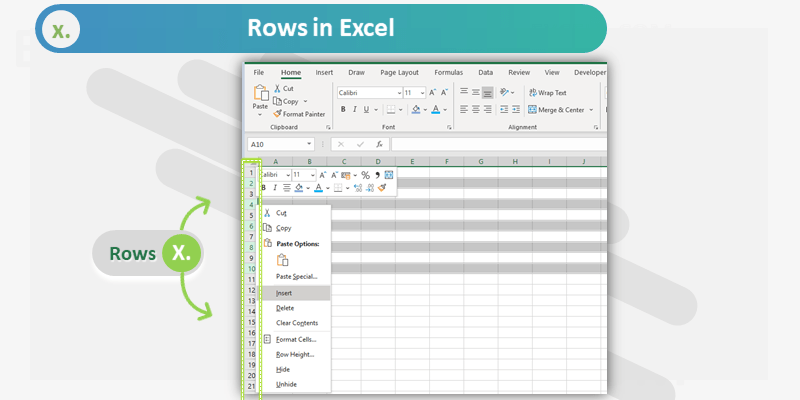Rows are Records in Excel Spreadsheet. Excel Spreadsheet is container in the Workbook, it is in Tabular Format with Rows (Horizontal records) and Columns (Vertical fields). Each row in a sheet represented with a unique row number, starts with row 1.

### Limitations:

Many Excel users raises these common question a about Excel Rows. How many rows in Excel Worksheet? Is there any limitation with Rows in Excel?

There are 1,048,576 rows in the latest version of Excel Application (from Office 2007 and higher).

The maximum Height of Excel Rows can set to 409 Points.

MS Office Version Row Limitation
Excel 2003 65536
Excel 2007 1048576
Excel 2010 1048576
Excel 2013 1048576
Excel 2016 1048576
Excel 2019 1048576

### Usage & Properties

Here are most common uses of Excel Rows in our daily Excel tasks. We can do the following tasks and change the values of below properties if required.

Properties Usage
Height We can increase and decrease the row height based on our requirement
Fill Color We can change the Interior Color if the Entire Row
Fonts We can select different font to a Row and format the font size, font name, font color, etc.
Hide/Unhide We can hide or unhide the rows if required
Group We can Group Specific number of Rows in Excel to Expand and Collapse.
Clear We can Clear Entire Row (range of rows) at a time
Insert We can Insert rows before or after a specif rows
Delete We can delete any specific rows if required

### Functions

Excel provided verity of built-in functions to deal with Rows. Here are the most commonly used Row Functions and Formulas.

Properties Usage
Row() Returns the Row number of the Cell
Row(address) Returns the Row number of the Given Address. Example: Row(A25) returns 25
Previous Row Row()-1, return the previous row of the Cell where the function is inserted. For example, if you insert this formula at A5, it returns 4.
Next Row Row()+1, return the next row of the Cell where the function is inserted. For example, if you insert this formula at A5, it returns 6.
Rows() Returns the Row Numbers
Rows(Range) Returns the Number of Rows in a given Range. For example, Rows(A2:C9) returns 9.
ROWS(1:1) Select the Entire first Row. For example, Sum(Rows(5:5)) returns total of Row 5 values.
Absolute  Reference We can use \$ symbol to make a row reference absolute. For example A\$5